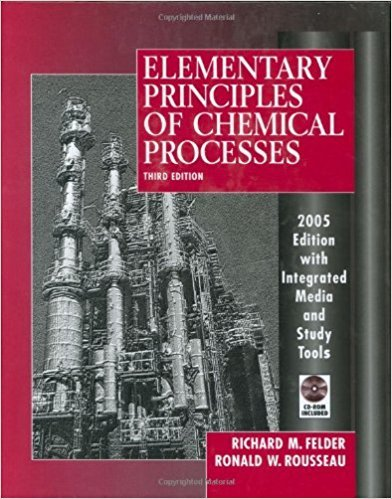×
Get Full Access to Elementary Principles Of Chemical Processes - 3 Edition - Chapter 5 - Problem 5.12
Get Full Access to Elementary Principles Of Chemical Processes - 3 Edition - Chapter 5 - Problem 5.12

×

# A gas cylinder filled with nitrogen at standardISBN: 9780471687573 143

## Solution for problem 5.12 Chapter 5

Elementary Principles of Chemical Processes | 3rd Edition

• Textbook Solutions
• 2901 Step-by-step solutions solved by professors and subject experts
• Get 24/7 help from StudySoup virtual teaching assistantsElementary Principles of Chemical Processes | 3rd Edition

4 5 1 248 Reviews
12
2
Problem 5.12

A gas cylinder filled with nitrogen at standard temperature and pressure has a mass of 37.289 g. The same container filled with carbon dioxide at STP has a mass of 37.440 g. When filled with an unknown gas at STP, the container mass is 37.062 g. Calculate the molecular weight of the unknown gas, and then state its probable identity

Step-by-Step Solution:

Problem 5.12A gas cylinder filled with nitrogen at standard temperature and pressure has a mass of 37.289g. The same container filled with carbon dioxide at STP has a mass of 37.440 g. When filledwith an unknown gas at STP, the container mass is 37.062 g. Calculate the molecular weight ofthe unknown gas, and then state its probable identity Step-by-step solution Step 1 of 2 ^To identify the gas present in the cylinder, first you need to determine the cylinder mass andthen take difference of the mass of cylinder filled with unknown gas and mass of empty cylinderwhich gives you the mass of unknown gas. Finally calculate the molecular weight of unknowngas from its mass and moles and obtain the identity of gas.The mass of cylinder filled with N at2TP is 37.289 g and that of cylinder filled with CO at STP 2is 37.440 g.This gives mass relations as follows: 37.289 g = m cylinderngas(28.02 g/mol) 37.440 g = m cylinderngas(44.01 g/mol)Here, m represents mass and n represents moles of gas in the cylinder.Use the above relations to solve for cylinder mass and moles of gas: ngas= 0.009443 mol mcylinder .024 g

Step 2 of 2

##### ISBN: 9780471687573

Unlock Textbook Solution